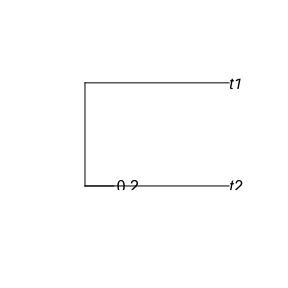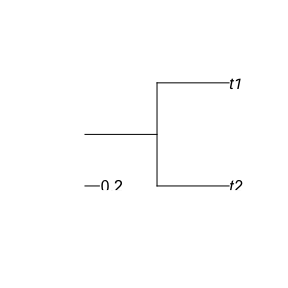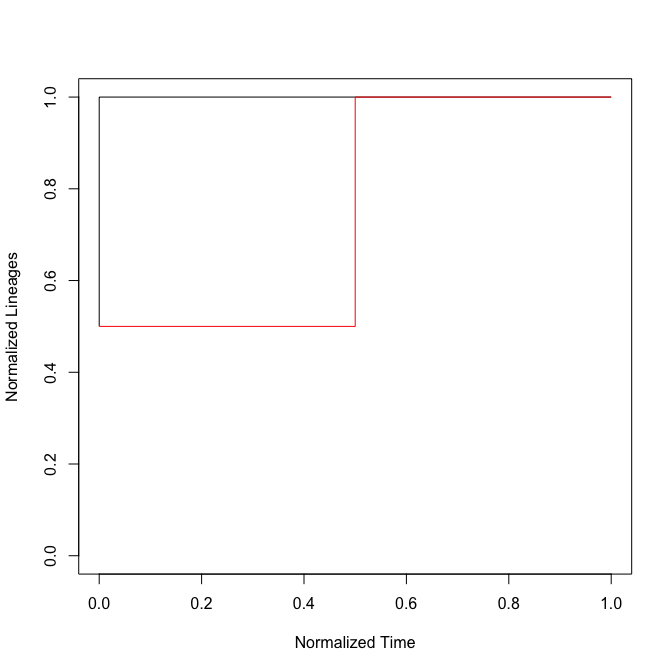Trees with a root edge

2020-01-09

This document intends to display a feature of the nLTT package: if a stem is added to a phylogeny, the begin of that stem is set as the most recent common ancestor.

Here I create a random phylogeny:

set.seed(42)
tree1 <- ape::rcoal(2)
tree1$edge.length <- tree1$edge.length / tree1$edge.length # nolint ape variable name I copy that same phylogeny and add a stem: tree2 <- tree1 tree2$root.edge <- 1 # nolint ape variable name

Here is how the stemless phylogeny looks like:

ape::plot.phylo(tree1, root.edge = TRUE)I set root.edge to TRUE, just show it is absent.

Here is how the phylogeny with stem looks like:

ape::plot.phylo(tree2, root.edge = TRUE)When overlaying their nLTT plots, one can expect two things:

• it is assumed that the phylogeny without a root is leading, and the other phylogeny has its root removed
• it is assumed that the phylogeny with a root is leading, and the other phylogeny is assumed to have a root of length zero

The nLTT package does the latter:

nLTT::nltt_plot(tree1, xlim = c(0, 1), ylim = c(0, 1))
nLTT::nltt_lines(tree2, col = "red")When looking at the nLTT plot, one can eyeball that the nLTT statistic (the surface between the two curves) is around about one quarter.

When calculating the nLTT statistic, one needs to explicitly set to take the stems into account:

print(nLTT::nLTTstat_exact(tree1, tree2, ignore_stem = FALSE))
##  0.25

When ignoring the stems, the phylogenies are equal, thus have an nLTT statistic of zero:

print(nLTT::nLTTstat_exact(tree1, tree2, ignore_stem = TRUE))
##  0